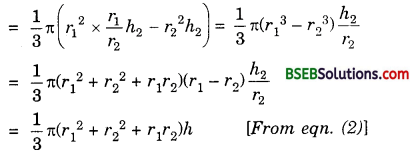# Bihar Board Class 10th Maths Solutions Chapter 13 Surface Areas and Volumes Ex 13.5

Bihar Board Class 10th Maths Solutions Chapter 13 Surface Areas and Volumes Ex 13.5 Textbook Questions and Answers.

## BSEB Bihar Board Class 10th Maths Solutions Chapter 13 Surface Areas and Volumes Ex 13.5Question 1.
A copper wire 3 mm in diameter is wound about a cylinder whose length is 12 cm, and diameter 10 cm, so as to cover the curved surface of the cylinder. Find the length and mass of the wire, assuming the density of copper to be 8.88 g per cm³.
Solution:
Curved surface area of the cylinder
= 2πrh = 2 x 3.14 x 5 x 12 cm²
= 120 x 3.14 cm²
Diameter i.e., width of the wire
= 3 mm = $$\frac { 3 }{ 10 }$$ cm
Let length of the wire be l cm.
So, area of the wire = l x $$\frac { 3 }{ 10 }$$ cm²
So, l x $$\frac { 3 }{ 10 }$$ = 120 x 3.14
or l = $$\frac{120 \times 3.14 \times 10}{3}$$ cm = 1256 cm
Now volume of wire = πr²h
= 3.14 x $$\frac { 3 }{ 20 }$$ x $$\frac { 3 }{ 20 }$$ x 1256 cm³
So, weight of wire = 3.14 x $$\frac { 9 }{ 400 }$$ x 1256 x 8.88 g
= 789.98 g (approx.)

Question 2.
A right triangle, whose sides are 3 cm and 4 cm (other than hypotenuse) is made to revolve about its hypotenuse. Find the volume and surface area of the double cone so formed. (Choose value of n as found appropriate.)
Solution:
In the right angled ∆ ABC,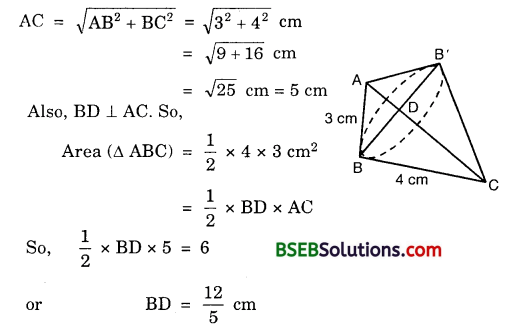So, the radius of the base for both the cones = $$\frac { 12 }{ 5 }$$ cm.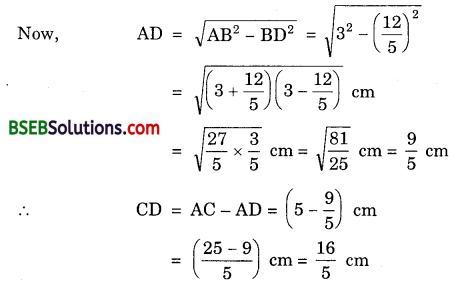∴ Volume of the cone ABB’
= $$\frac { 1 }{ 3 }$$π.BD².AD
= $$\frac { 1 }{ 3 }$$ x $$\frac { 22 }{ 7 }$$ x $$\frac { 144 }{ 25 }$$ x $$\frac { 9 }{ 5 }$$cm³ = $$\frac { 9504 }{ 875 }$$ cm³
Volume of the cone CBB’
= $$\frac { 1 }{ 3 }$$π.BD².CD
= $$\frac { 1 }{ 3 }$$ x $$\frac { 22 }{ 7 }$$ x $$\frac { 144 }{ 25 }$$ x $$\frac { 16 }{ 5 }$$cm³ = $$\frac { 16896 }{ 875 }$$ cm³
∴Volume of the double cone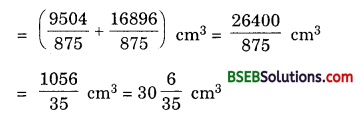Curved surface area of the cone ABB’
= π.BD.CD
= $$\frac { 22 }{ 7 }$$ x $$\frac { 12 }{ 5 }$$ x 3 cm² = $$\frac { 792 }{ 35 }$$ cm²
Curved surface area of the cone CBB’
= π.BD.CD
= $$\frac { 22 }{ 7 }$$ x $$\frac { 12 }{ 5 }$$ x 4 cm² = $$\frac { 1056 }{ 35 }$$ cm²
∴ Surface area of the double cone =
= $$\left(\frac{792}{35}+\frac{1056}{35}\right)$$ cm²
= $$\frac { 1848 }{ 35 }$$ cm²
= 52.8 cm²Question 3.
A cistern, internally measuring 150 cm x 120 cm x 110 cm, has 129600 cm³ of water in it. Porous bricks are placed in the water until the cistern is full of the brim. Each brick absorbs one-seventeenth of its own volume of water. How many bricks can be put in without overflowing the water, each brick being 22.5 cm x 7.5 cm x 6.5 cm?
Solution:
Volume of the cistern = 150 cm x 120 cm x 110 cm = 1980000 cm³
Let n bricks can be put in the cistern without over flowing water
Then, volume of n bricks = n x (22.5 x 7.5 x 6.5) cm³ = 1096.875 x n cm³
Volume of water absorbed by n bricks
= $$\frac { 1 }{ 17 }$$ x 1096.875 x n cm³
= 64.52 x n cm³
∴1096.875 x n + 129600 – 64.52 x n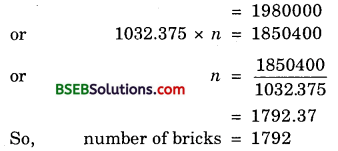Question 4.
In one fortnight of a given month, there was a rainfall of 10 cm in a river valley. If the area of the valley is 97280 km2, show that the total rainfall was approximately equivalent to the addition to the normal water of three rivers each 1072 km long, 75 m wide and 3 m deep.
Solution:
Volume of water in the 3 riversSo, the addition of water in the rivers is not equivalent to the rainfall.

Question 5.
An oil funnel of tin sheet consists of a 10 cm long cylindrical portion attached to a frustum of a cone. If the total height is 22 cm, diameter of the cylindrical portion is 8 cm and the diameter of the top of the funnel is 18 cm, find the area of the tin sheet required to make the funnel (see figure).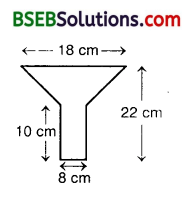Solution:
The diameter of the cylindrical portion is 8 cm.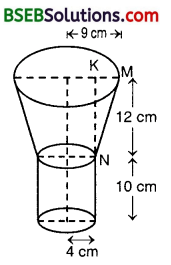Radius of top portion = 9 cm
Here, r = 4 cm, R = 9 cm, h = 12 cm
KM = (9-4) cm = 5 cm
∴ NM² = l² = KM² + KN2
= 5² + 12²
= 25 + 144 = 169
So, l = $$\sqrt{169}$$ cm = 13 cm
Now, the tin required will be equal to the total surface area of the figure.
Area of the tin required = Curved surface area of the cylinder + Curved surface area of the frustumQuestion 6.
Derive the formula for the curved surface area and total surface area of the frustum of a cone, given to you in Section 13.5, using the symbols as explained.
Solution:
Curved surface area of the frustum RPQS = Curved surface area of the right circular cpne OPQ – Curved surface area of the right circular cone ORS
= πr1l1 – πr2l2 … (1)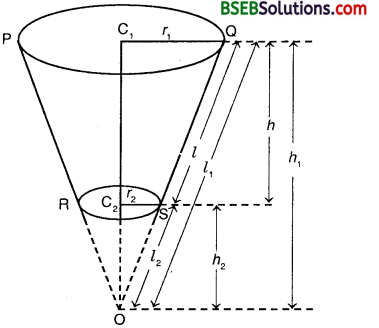Now, since ∆ OC1Q and ∆ OC2S are similar, thereforePutting the value of l1 in terms of r1, r2 and l2 in equation (1), we have :
Curved surface area of the frustum∴Total surface area of the frustum
= Curved surface area of the frustum + πr1² + πr2²
= π(r1 + r2)l + πr1² + πr2²
= π[(r1 + r2)l + r1² + r2²]Question 7.
Dev ive the formula for the volume of the frustum of a cone, given to you in Section 13.5, using the symbols as explained.
Solution:
Volume of the frustum RPQS = Volume of the right circular cone OPQ – Volume of the right circular cone ORS
= $$\frac { 1 }{ 3 }$$πr1²h1 – $$\frac { 1 }{ 3 }$$πr2²h2
= $$\frac { 1 }{ 3 }$$π(r1²h1 – r2²h2) … (1)
Now, since ∆ OC1Q and ∆ OC2S are similar, therefore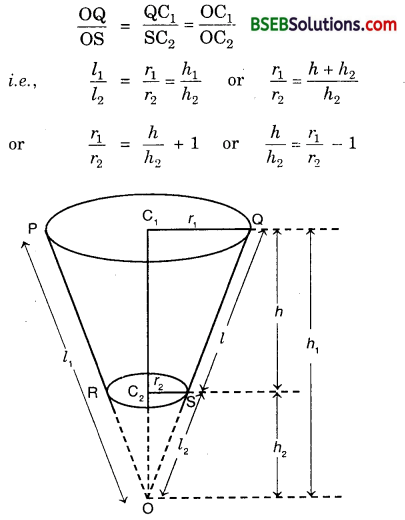or h1 = $$\left(\frac{r_{1}}{r_{2}}-1\right)$$ h2 = $$\left(\frac{r_{1}-r_{2}}{r_{2}}\right)$$h2 … (2)
Putting the value of in terms of rr r2 and h2 in eqn. (1), we have :
Volume of the frustum